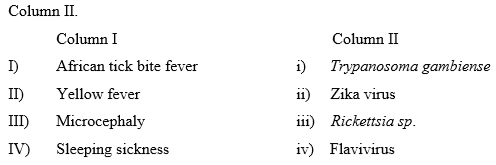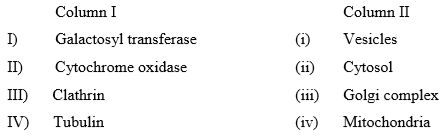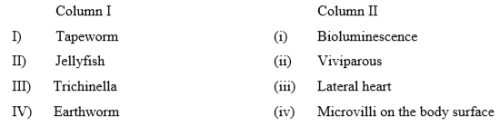Courses

# Life Sciences - (XL) 2018 GATE Paper (Practice Test - 5)

## 20 Questions MCQ Test GATE Past Year Papers for Practice (All Branches) | Life Sciences - (XL) 2018 GATE Paper (Practice Test - 5)

Description
This mock test of Life Sciences - (XL) 2018 GATE Paper (Practice Test - 5) for GATE helps you for every GATE entrance exam. This contains 20 Multiple Choice Questions for GATE Life Sciences - (XL) 2018 GATE Paper (Practice Test - 5) (mcq) to study with solutions a complete question bank. The solved questions answers in this Life Sciences - (XL) 2018 GATE Paper (Practice Test - 5) quiz give you a good mix of easy questions and tough questions. GATE students definitely take this Life Sciences - (XL) 2018 GATE Paper (Practice Test - 5) exercise for a better result in the exam. You can find other Life Sciences - (XL) 2018 GATE Paper (Practice Test - 5) extra questions, long questions & short questions for GATE on EduRev as well by searching above.
QUESTION: 1

Solution:
QUESTION: 2

Solution:
QUESTION: 3

### In a chemical reaction where the substrate and product are in equilibrium in solution, what will occur if an enzyme is added?

Solution:
QUESTION: 4

Tay-Sachs disease is a human genetic disorder that is associated with defects in which ONE of the following cellular organelles?

Solution:
QUESTION: 5

Increase in the existent population of grey peppered moth, Biston betularia, during industrial revolution in Britain is an example of which ONE of the following evolutionary processes?

Solution:
QUESTION: 6

Which ONE of the following is NOT a characteristic of a cancer cell?

Solution:
QUESTION: 7

Cardiac and cerebral tissues are derived from the following germ layers respectively

Solution:
QUESTION: 8

An animal’s ability to escape from a predator by using the explored knowledge of home area is an example of

Solution:
QUESTION: 9

Bowman’s capsules are present in which ONE of the following organs/ tissues?

Solution:
QUESTION: 10

Which ONE of the following is the primary function of lung surfactants?

Solution:
QUESTION: 11

Match the disorders/diseases listed in Column I to their respective causative agents listed in Column II.Solution:
QUESTION: 12

Glucose monomers are joined together by glycosidic linkages to form a cellulose polymer. During this process, changes in the free energy, total energy, and entropy respectively are represented correctly by which ONE of the following options?

Solution:
QUESTION: 13

In Drosophila melanogaster, a mutation in Ultrabithorax which defines the third segment of the thorax or T3 leads to development of four winged flies, as the halteres develop into a second pair of wings. Which ONE of the following phenotypes in fly will result from overexpression of Ultrabithorax in the second thoracic segment?

Solution:
QUESTION: 14

Which ONE of the following is TRUE in case of respiratory acidosis?

Solution:
QUESTION: 15

Match the proteins / molecules listed in column I with the cellular location mentioned in the column IISolution:
QUESTION: 16

In an experiment, nucleus from a Drosophila oocyte was transplanted into the anterior part of another oocyte, at a region opposite to the existing nucleus. Which ONE of the following phenotypes will the developing egg show?

Solution:
QUESTION: 17

Match the organisms listed in Column I with the features listed in Column IISolution:
QUESTION: 18

Which ONE of the following statements is NOT part of the classical Darwinian theory of evolution by natural selection?

Solution:
QUESTION: 19

A population of rabbits was determined to have a birth rate of 200 and mortality rate of 50 per year. If the initial population size is 4000 individuals, after 2 years of non-interfered breeding the final population size will be _______________.

Solution:
QUESTION: 20

In a population which is in Hardy-Weinberg equilibrium, the frequency of occurrence of a disorder caused by recessive allele (q) is 1 in 1100. The frequency of heterozygotes in the population will be ______________. (Give the answer to three decimal places).

Solution: